# C186 – Pressure Calculation – Absolute and Gauge

## Description

This calculates pressure generated either using a pressure balance (dead weight tester) as the standard or a digital pressure indicator or gauge. Where appropriate the equation can be solved for either pressure or mass to generate pressure.

Kelton calculation reference C186

KIMS calculation reference K200

FLOCALC calculation reference F018

This replaces calculation C130/K120

## Options

### Pressure Mode

• Absolute

This option enables the use of a reference pressure, for example, absolute pressure indicating instruments can be calibrated by generating gauge pressure using a dead weight pressure and adding a reference pressure which is equal to barometric pressure. If this is selected make sure that pressure input is set to use absolute pressure units.

• Gauge

If gauge mode is selected the reference pressure is taken to be 1 atmosphere.

### Reference standard

• Dead weight tester – ‘mass and effective area’ method

Use this option if you are generating pressure using a dead weight tester (pressure balance) which is calibrated to give values for mass and effective area.

• Dead weight tester – ‘Kn’ method

Use this option if you are generating pressure using a dead weight tester (pressure balance) which is calibrated to give the mass pressure ratio Kn.

• Dead weight tester – ‘pressure’ method

Use this option if you are generating pressure using a dead weight tester (pressure balance) using a nominal value with corrections.

• Digital pressure indicator or gauge.

Use this option if you are generating pressure using other methods, for example, a digital pressure indicator or gauge.

### Output Type

• Digital

For this option the output is in pressure units

• Analogue

Fot this option the output is converted to a voltage or current.

### Calibration Correction

• Apply

Select this option to apply a correction to account for values obtained through laboratory calibration, for example a foot-print calibration of a differential pressure transmitter.

### Correct For

If selected, the head correction is added to the calculated pressure output.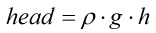Where ρ = The density of the pressure transmitting fluid g = The local value for acceleration due to gravity h = The height of the tester above the instrument being calibrated
• Temperature

If selected, allows the user to input values for Piston/Cylinder temperature, Calibration temperature and Temperature coefficient.

• Density of air

If not selected, the default value is 1.2kgm-3.

• Density of mass

If not selected, the default value is 8000 kgm-3.

• Surface Tension

If not selected, the default value is 0 N.

• Buoyancy

If not selected, the default value is 0 N.

• Gravity

If not selected, the local gravity is taken as 9.80665ms-2.

## Calculations

### Dead Weight Tester – ‘Mass and Effective Area’ Method

• Solving for Pressure

This equation calculates the pressure generated by loading a piston with mass.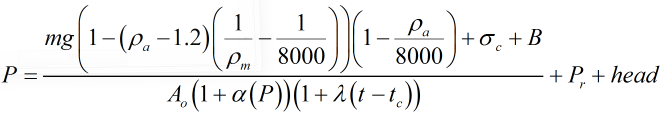• Solving for Mass

This equation calculates the applied mass required to generate pressure.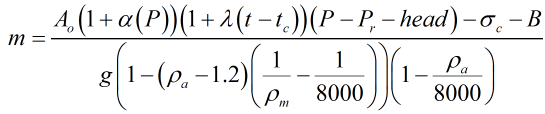Where m = Mass Pr = Residual Pressure; this will typically be barometric pressure or the bell jar pressure for an absolute DWT P = Pressure ρa = Density of Air ρm = Density of the Mass σc = Force due to Surface Tension B = Force due to Piston Buoyancy gl = Local Gravity Ao = Effective Area α = Pressure coefficient λ = Temperature coefficient (the combined temperature coefficient of the piston cylinder unit ap + ac) tc = Calibration temperature t = PC temperature

### Dead Weight Tester – ‘Kn’ Method

• Solving for Pressure

This equation calculates the pressure generated by loading a piston with mass.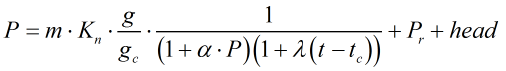• Solving for Mass

This equation calculates the applied mass required to generate pressure.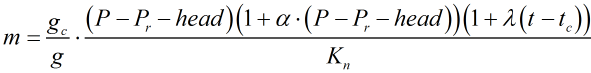Where m = Mass Pr = Residual Pressure P = Pressure gl = Local Gravity gc = Calibration reference gravity Kn = Pressure ratio α = Pressure Coefficient λ = Temperature Coefficient (the combined temperature coefficient of the piston cylinder unit ap + ac) tc = Calibration Temperature t = PC Temperature

### Dead Weight Tester – ‘Pressure’ method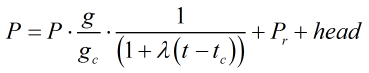Where Pr = Residual pressure gl = Local gravity gc = Calibration reference gravity λ = Temperature coefficient (the combined temperature coefficient of the piston cylinder unit ap + ac) tc = Calibration temperature t = PC temperature

### Digital Pressure Indicator or Gauge

For this option the generated pressure is taken to be that which is indicated on the standard corrected for head where applicable.

Back